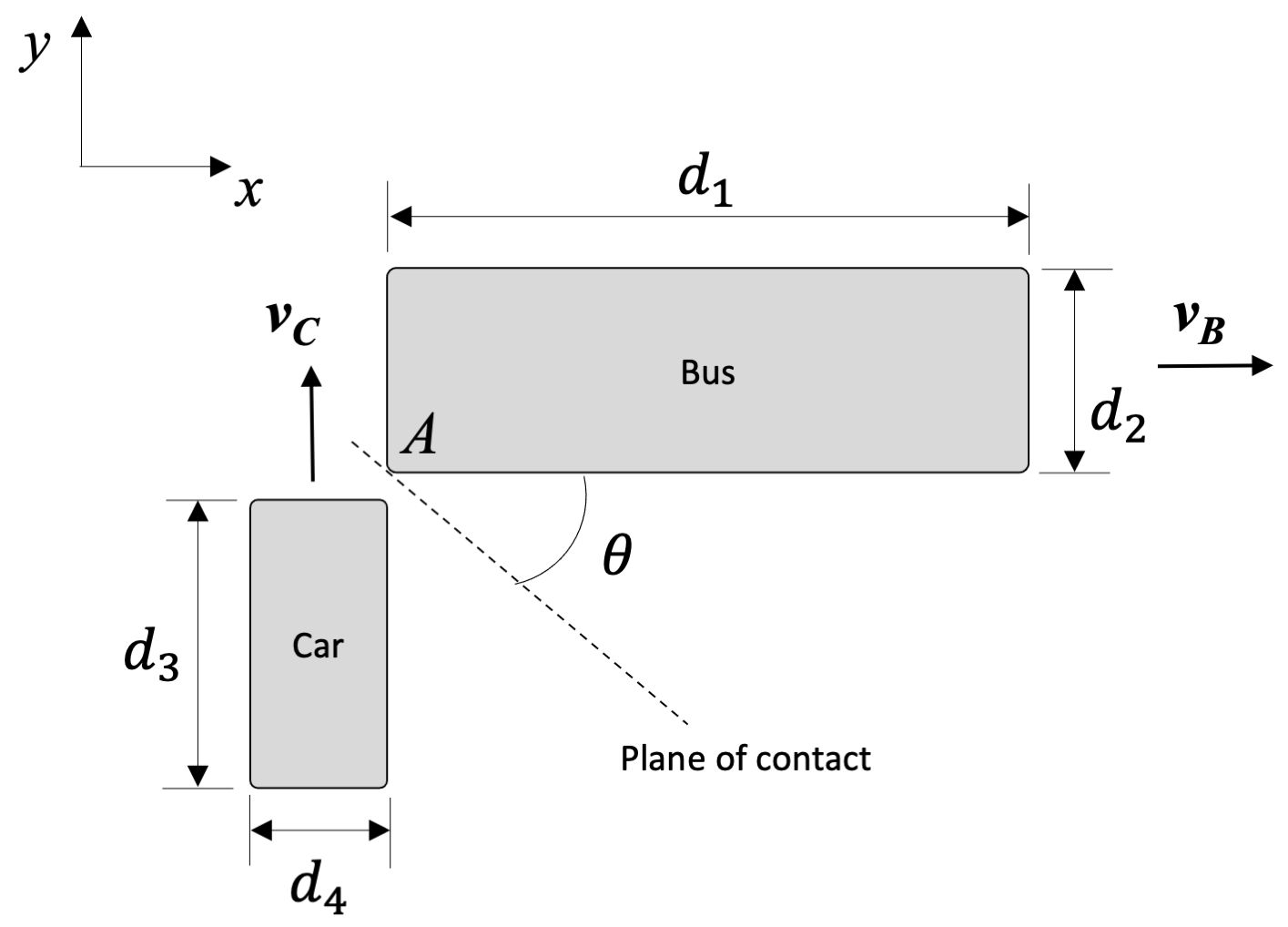﻿ Mechanics Map - Free Rigid Body Collisions

# Free Rigid Body Collisions

A free rigid body collision is an instance where two non-anchored or movable bodies impact one another, and we are treating at least one of those bodies as a rigid body where rotation can occur.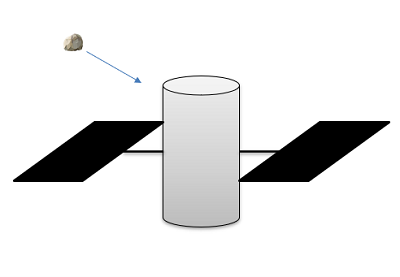The meteoroid impacting this satellite is an example of a free rigid body collision. The collision will result in a change in velocity and angular velocity for the satellite.

## Rigid Body Inelastic Impacts

We will begin our analysis with inelastic impacts, where the bodies stick together after impact. In these systems we will first recognize that the large internal impulsive forces between the bodies colliding dwarf any external forces at the time of collision. Though the linear momentum of each body individually will change drastically, the linear momentum of the system as a whole will not change. Assuming we call our two bodies A and B and we take the velocities at the center of mass (G), we arrive at the vector equation below.

 $m_{A} \vec{v_{GAi}}+m_{B} \vec{v_{GBi}}= (m_{A}+m_{B}) \vec{v_{GABf}}$

Furthermore, breaking this vector equation down into x and y components, we will arrive at the following set of scalar equations.

 $m_{A} v_{GAix}+m_{B} v_{GBix}= (m_{A}+m_{B}) v_{GABfx}$ $m_{A} v_{GAiy}+m_{B} v_{GBiy}= (m_{A}+m_{B}) v_{GABfy}$

In addition to the linear momentum being conserved, the angular momentum is also conserved for the system as a whole, as the large impact's angular impulse is internal. The angular momentum can be taken about any point, so long as we are consistent throughout the equation. For more information on calculating the angular momentum about various points, consult the prior sections.

 $angular\ momentum\ initial = angular\ momentum\ final$

## Rigid Body Elastic and Semi-Elastic Impacts

In an elastic or semi-elastic impact the bodies do not stick together after impact and will have separate linear and angular velocities post impact. With potential linear velocities in the x and y directions for each body, as well as separate angular velocities post impact, we will potentially have six unknowns to solve for. This also means that we will need six equations to solve for all of those unknowns.

The first three equations will be the same as with the inelastic impact. Both linear momentum and angular momentum will be conserved for the system as a whole.

 $m_{A} v_{GAix}+m_{B} v_{GBix}= m_{A} v_{GAfx}+m_{B} v_{GBfx}$ $m_{A} v_{GAiy}+m_{B} v_{GBiy}= m_{A} v_{GAfy}+m_{B} v_{GBfy}$ $angular\ momentum\ initial = angular\ momentum\ final$

As before, the linear velocities need to be taken at the center of mass (G), and the angular momentum can be taken about any point so long as we are consistent with the point we are taking it about throughout the equation.

To add to those three equations, as with rigid body surface impacts, we can assume the angular momentum of each body individually is conserved about the point of impact (P). This is because there will be no impulse angular impulse during the collision about this point in particular. As this point is almost always not at the center of mass of either body, we wind up with the following two equations.

 $I_{GA} * \vec{\omega_{Ai}} + \left( \vec{r}_{G_A/P} \times m_A \vec{v_{GBi}} \right) = I_{GA} * \vec{\omega_{Af}} + \left( \vec{r}_{G_A/P} \times m_A \vec{v_{GAf}} \right)$ $I_{GB} * \vec{\omega_{Bi}} + \left( \vec{r}_{G_B/P} \times m_B \vec{v_{GBi}} \right) = I_{GB} * \vec{\omega_{Bf}} + \left( \vec{r}_{G_B/P} \times m_A \vec{v_{GBf}} \right)$

Finally, we can also use the coefficient of restitution equation, at least assuming we know what this coefficient is. For this equation, it will be important to look at the velocities at the point of impact (P) and to specifically look at the velocities normal to the plane of impact, similar to what we did with particles.

 $e=-\frac{v_{APnf} - v_{BPnf}}{v_{APni} - v_{BPni}}$

Along with kinematic relationships, combinations of the above equations and strategies can allow us to analyze post-impact motion of the objects. This is useful in areas such as accident reconstruction, where engineers determine what happened in a vehicular crash.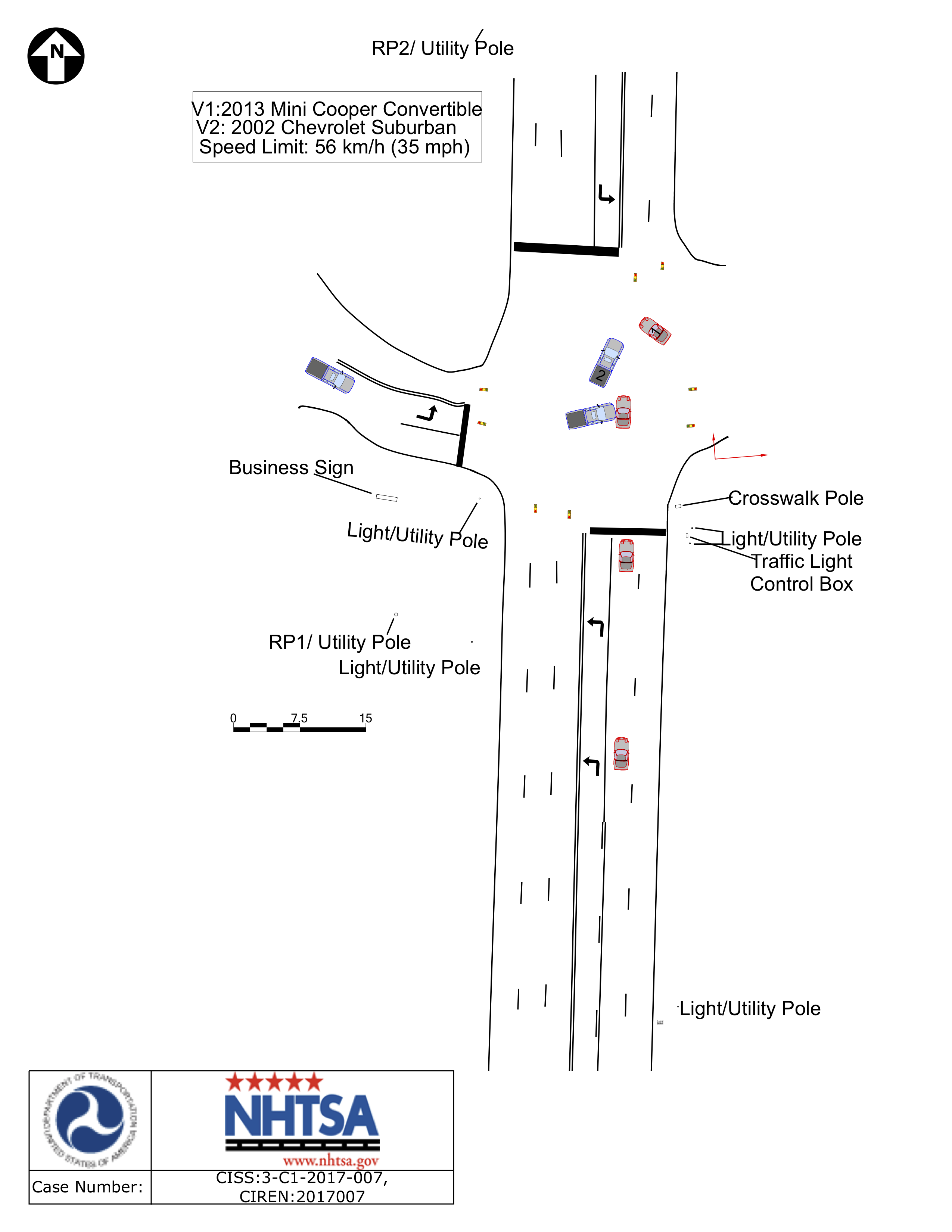The diagram shows the result of a vehicle crash investigation where engineers used techniques from free rigid body collisions to determine the incoming vehicle velocities based on information from the scene, including the final positions of the vehicles. Public Domain image from (NHTSA CIREN case 21).

## Worked Problems:

### Question 1:

A space telescope with a mass of 20,000 kg (approximate as a cylinder with a length of 10 m and a diameter of 2 m) is struck on one end with a micrometeorite with a mass .003 kg and a velocity of 10,000 m/s (model as a point mass). Assuming the micrometeorite becomes lodged in to space telescope, What is the expected velocity and angular velocity for the space telescope after impact?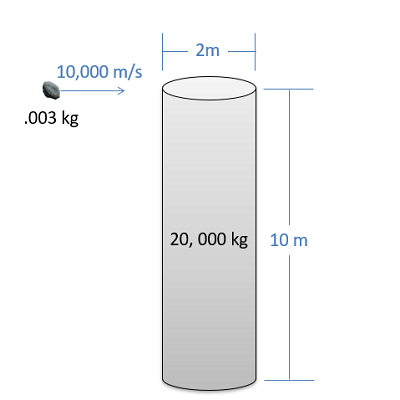### Question 2:

A Rube Goldberg machine utilizes a pool ball and a slender 2 m long rod. If the pool ball has a mass m_ball=0.16 kg while the rod has mass m_rod=1 kg, determine the angular velocity of the rod when the pool ball strikes it at v=3 m/s. The pool ball has radius r=0.025 m and the coefficient of restitution is e=0.8. The rod hits the pool ball at the same height as the ball’s center of gravity.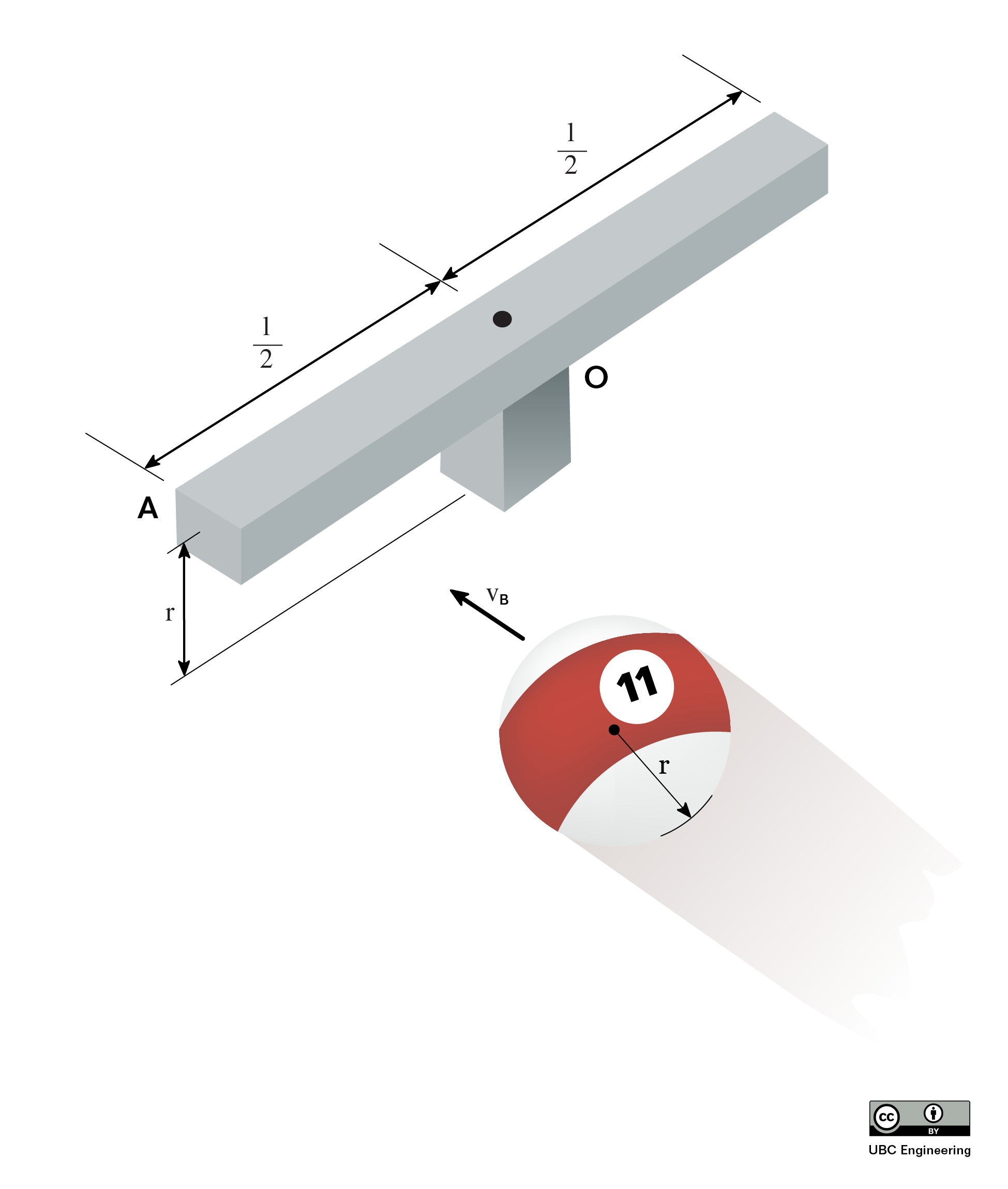### Question 3:

A rod (mR = 5 kg, L = 3 m) rests on a frictionless surface. A ball (mB = 1 kg) with an initial velocity vB1 = 12 m/s in the direction shown impacts the rod at P. Find the rod’s angular velocity, ωR2, and linear velocity of the center of gravity, vG2, immediately after impact if b = 1 m. Assume e = 0.6.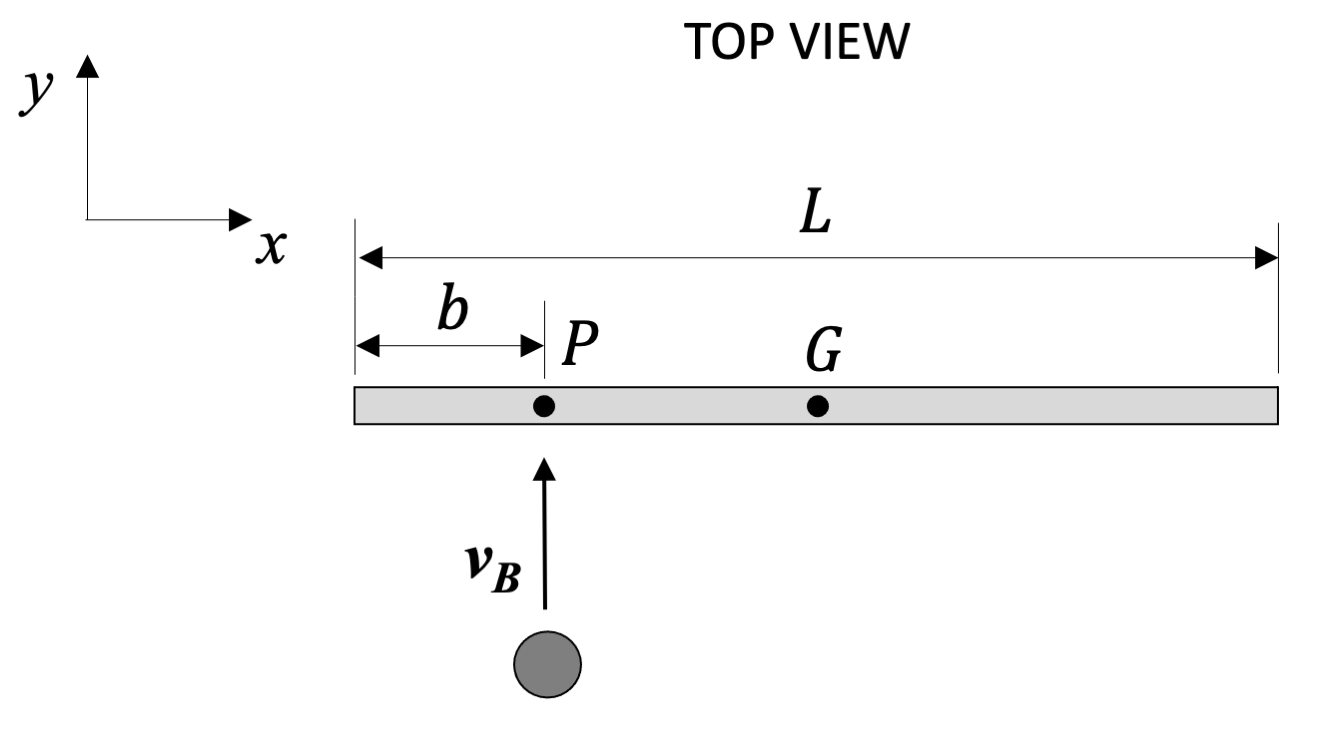### Question 4:

A car (mC = 2000 kg, d3 = 3 m, d4 = 1.5 m) is driving on an icy road (assume frictionless road surface). It is unable to stop at an intersection and impacts a bus (mB = 8000 kg, d1 = 9 m, d2 = 2.5 m). The initial vehicle velocities are vC1 = 5 m/s and vB1 = 10 m/s. Assume the impact is such that the vehicles corners contact at A, and the plane of contact is θ = 0o. Find the angular velocity of each vehicle just after impact, ωC2 and ωB2, if the impact is completely plastic at A. Assume the car and the bus can be treated as constant density objects.